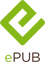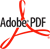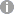# Advanced Data Analysis and Modelling in Chemical Engineering

Elsevier (Verlag)
• 1. Auflage
• |
• erschienen am 23. August 2016
• |
• 414 Seiten

 E-Book | ePUB mit Adobe DRM | Systemvoraussetzungen E-Book | PDF mit Adobe DRM | Systemvoraussetzungen
978-0-444-59484-6 (ISBN)

Advanced Data Analysis and Modeling in Chemical Engineering provides the mathematical foundations of different areas of chemical engineering and describes typical applications. The book presents the key areas of chemical engineering, their mathematical foundations, and corresponding modeling techniques.

Modern industrial production is based on solid scientific methods, many of which are part of chemical engineering. To produce new substances or materials, engineers must devise special reactors and procedures, while also observing stringent safety requirements and striving to optimize the efficiency jointly in economic and ecological terms. In chemical engineering, mathematical methods are considered to be driving forces of many innovations in material design and process development.

• Presents the main mathematical problems and models of chemical engineering and provides the reader with contemporary methods and tools to solve them
• Summarizes in a clear and straightforward way, the contemporary trends in the interaction between mathematics and chemical engineering vital to chemical engineers in their daily work
• Includes classical analytical methods, computational methods, and methods of symbolic computation
• Covers the latest cutting edge computational methods, like symbolic computational methods

Denis Constales is an applied mathematician who has been working in chemical engineering and statistics for the past 12 years, specializing in diffusion problems, parameter estimation and inverse problems, chemical kinetics, reaction mechanism identification, and nearly all aspects of the Temporal Analysis of Products method.
 Reihe: Sprache: Englisch Verlagsort: Oxford | Niederlande Verlagsgruppe: Elsevier Science Dateigröße: 24,15 MB Schlagworte: ISBN-13: 978-0-444-59484-6 (9780444594846) ISBN-10: 0-444-59484-1 (0444594841)weitere Ausgaben werden ermittelt
• Front Cover
• Advanced Data Analysis and Modelling in Chemical Engineering
• Contents
• Preface
• Our Team
• Chapter 1: Introduction
• 1.1. Chemistry and Mathematics: Why They Need Each Other
• 1.2. Chemistry and Mathematics: Historical Aspects
• 1.3. Chemistry and Mathematics: New Trends
• 1.4. Structure of This Book and Its Building Blocks
• Chapter 2: Chemical Composition and Structure: Linear Algebra
• 2.1. Introduction
• 2.2. The Molecular Matrix and Augmented Molecular Matrix
• 2.2.1. The Molecular Matrix
• 2.2.2. Application of the Molecular Matrix to Element Balances
• 2.2.3. Remarks
• 2.3. The Stoichiometric Matrix
• 2.3.1. Application of the Stoichiometric Matrix to Reactions
• 2.3.2. The Augmented Stoichiometric Matrix
• 2.3.2.1. How to find the key and nonkey reactions
• 2.3.2.2. Enthalpy change and equilibrium coefficients
• 2.4. Horiuti Numbers
• 2.5. Summary
• Appendix. The RREF in Python
• Chapter 3: Complex Reactions: Kinetics and Mechanisms - Ordinary Differential Equations - Graph Theory
• 3.1. Primary Analysis of Kinetic Data
• 3.1.1. Introduction
• 3.1.2. Types of Reactors for Kinetic Experiments
• 3.1.3. Requirements of the Experimental Information
• 3.2. Material Balances: Extracting the Net Rate of Production
• 3.2.1. Transport in Reactors
• 3.2.2. Change Due to the Chemical Reaction-Conversion
• 3.2.3. Ideal Reactors (Homogeneous)
• 3.2.3.1. The batch reactor
• 3.2.3.2. The continuous stirred-tank reactor
• 3.2.3.3. The plug-flow reactor
• 3.2.4. Ideal Reactors With Solid Catalyst
• 3.2.4.1. The batch reactor
• 3.2.4.2. The continuous stirred-tank reactor
• 3.2.4.3. The plug-flow reactor
• 3.2.4.4. The temporal analysis of products reactor
• 3.2.5. Summary
• 3.3. Stoichiometry: Extracting the Reaction Rate From the Net Rate of Production
• 3.3.1. Complexity and Mechanism: Definition of Elementary Reactions
• 3.3.2. Homogeneous Reactions: Mass-Action Law
• 3.3.3. Heterogeneous Reactions
• 3.3.4. Rate Expressions for Single Reactions: Stoichiometry
• 3.3.5. Relationships Between Reaction Rate and Net Rate of Production
• 3.4. Distinguishing Kinetic Dependences Based on Patterns and Fingerprints
• 3.4.1. Single Reactions
• 3.4.1.1. Irreversible reaction
• 3.4.1.2. Reversible reaction
• 3.4.1.3. Reversible reaction with Langmuir-Hinshelwood-Hougen-Watson kinetics
• 3.4.2. Distinguishing Between Single and Multiple Reactions
• 3.4.3. Distinguishing Between Elementary and Complex Reactions
• 3.4.4. Summary of Strategy
• 3.5. Ordinary Differential Equations
• 3.6. Graph Theory in Chemical Kinetics and Chemical Engineering
• 3.6.1. Introduction
• 3.6.2. Derivation of a Steady-State Equation for a Complex Reaction Using Graph Theory
• 3.6.2.1. Example: Two-step Temkin-Boudart mechanism
• 3.6.2.2. General steady-state kinetic equations
• 3.6.2.3. Trees in graph theory
• 3.6.3. Derivation of Steady-State Kinetic Equations for Multiroute Mechanisms: Kinetic Coupling
• 3.6.3.1. Cycles having a common intermediate
• 3.6.3.2. Cycles having a common step
• 3.6.2.3. Cycles having two common steps
• 3.6.2.4. Different types of coupling between cycles
• 3.6.4. Bipartite Graphs of Complex Reactions
• 3.6.5. Graphs for Analyzing Relaxation: General Form of the Characteristic Polynomial
• Chapter 4: Physicochemical Principles of Simplification of Complex Models
• Superscripts
• 4.1. Introduction
• 4.2. Physicochemical Assumptions
• 4.2.1. Assumptions on Substances
• 4.2.2. Assumptions on Reactions and Their Parameters
• 4.2.3. Assumptions on Transport-Reaction Characteristics
• 4.2.4. Assumptions on Experimental Procedures
• 4.2.5. Combining Assumptions
• 4.3. Mathematical Concepts of Simplification in Chemical Kinetics
• 4.3.1. Introduction
• 4.3.2. Simplification Based on Abundance
• 4.3.3. Rate-Limiting Step Approximation
• 4.3.4. Quasi-equilibrium Approximation
• 4.3.5. Quasi-Steady-State Approximation
• 4.3.6. Mathematical Status of the Quasi-Steady-State Approximation
• 4.3.7. Simplifications and Experimental Observations
• 4.3.8. Lumping Analysis
• Chapter 5: Physicochemical Devices and Reactors
• 5.1. Introduction
• 5.2. Basic Equations of Diffusion Systems
• 5.2.1. Ficks Laws
• 5.2.2. Einsteins Mobility and Stokes Friction
• 5.2.3. Knudsen Diffusion and Diffusion in Nonporous Solids
• 5.2.4. Onsagers Approach
• 5.2.5. Teorells Approach
• 5.2.6. Nonlinear Diffusion Models
• 5.3. Temporal Analysis of Products Reactor: A Basic Reactor-Diffusion System
• 5.3.1. Introduction
• 5.3.2. Typical TAP Reactor Configurations
• 5.3.2.1. Three-zone TAP reactor
• 5.3.2.2. Thin-zone TAP reactor
• 5.3.2.3. Single-particle TAP reactor
• 5.3.3. Main Principles of TAP
• 5.4. TAP Modeling on the Laplace and Time Domain: Theory
• 5.4.1. The Laplace Transform
• 5.4.2. Key Results
• 5.5. TAP Modeling on the Laplace and Time Domain: Examples
• 5.5.1. Moment Definitions
• 5.5.2. One-Zone Reactor, Diffusion Only
• 5.5.3. One-Zone Reactor, Irreversible Adsorption
• 5.5.4. One-Zone Reactor, Reversible Adsorption
• 5.5.5. Three-Zone Reactor
• 5.5.6. Reactive Thin Zones
• 5.5.7. Other Thin Zones
• 5.5.7.1. Capacity and resistor zones
• 5.5.7.2. Generalized boundary conditions
• 5.6. Multiresponse TAP Theory
• 5.7. The Y Procedure: Inverse Problem Solving in TAP Reactors
• 5.8. Two- and Three-Dimensional Modeling
• 5.8.1. The Two-Dimensional Case
• 5.8.2. The Three-Dimensional Case
• 5.8.3. Summary
• 5.8.4. Radial Diffusion in Two and Three Dimensions
• 5.9. TAP Variations
• 5.9.1. Infinite Exit Zone
• 5.9.2. Internal Injection
• 5.9.3. Nonideal Plug-Flow Reactor via Transfer Matrix
• 5.10. Piecewise Linear Characteristics
• 5.10.1. Introduction
• 5.10.2. Conical Reactors
• 5.10.3. Surface Diffusion
• 5.10.4. Independence of the Final Profile of Catalyst Surface Coverage
• 5.11. First- and Second-Order Nonideality Corrections in the Modeling of Thin-Zone TAP Reactors
• 5.11.1. Thin-Zone TAP Reactor
• 5.11.2. Thin-Sandwiched TAP Reactor Matrix Modeling
• Chapter 6: Thermodynamics
• 6.1. Introduction
• 6.1.1. Thermodynamic Systems
• 6.1.2. Thermodynamic State Functions
• 6.1.3. Steady State and Equilibrium
• 6.1.4. Reversibility and Irreversibility
• 6.1.5. Equilibrium and Principle of Detailed Balance
• 6.2. Chemical Equilibrium and Optimum
• 6.2.1. Introduction
• 6.2.2. Le Chatelier's Principle
• 6.2.3. Relation Between Equilibrium and Optimum Regime
• 6.2.3.1. Equilibrium conversion
• 6.2.3.2. Optimum conversion in a continuous plug-flow reactor
• 6.2.3.3. Relationships between equilibrium and optimum conversions
• 6.2.3.4. Continuous stirred-tank reactor
• 6.2.4. Summary
• 6.3. Is It Possible to Overshoot an Equilibrium?
• 6.3.1. Introduction
• 6.3.2. Mass-Action Law and Overshooting an Equilibrium
• 6.3.3. Example of Equilibrium Overshoot: Isomerization
• 6.3.4. Requirements Related to the Mechanism of a Complex Chemical Reaction
• 6.4. Equilibrium Relationships for Nonequilibrium Chemical Dependences
• 6.4.1. Introduction
• 6.4.2. Dual Experiments
• 6.5. Generalization. Symmetry Relations and Principle of Detailed Balance
• 6.5.1. Introduction
• 6.5.2. Symmetry Between Observables and Initial Data
• 6.5.3. Experimental Evidence
• 6.5.4. Concluding Remarks
• 6.6. Predicting Kinetic Dependences Based on Symmetry and Balance
• 6.6.1. Introduction
• 6.6.2. Theoretical Model
• 6.6.3. Matrix of Experimental Curves
• 6.6.4. General Considerations
• 6.6.5. Checking Model Completeness
• 6.6.5.1. Closing the mass balance
• 6.6.5.2. Completeness test
• 6.6.6. Concluding Remarks
• 6.7. Symmetry Relations for Nonlinear Reactions
• 6.7.1. Introduction
• 6.7.2. Single Reversible Reactions
• 6.7.2.1. First-first order: A?B
• 6.7.2.2. Second-first order: 2A?B
• 6.7.2.3. Second-second order: 2A?2B
• 6.7.2.4. Second-first order: A+B?C
• 6.7.2.5. Second-second order: A+B?2C
• 6.7.2.6. Second-second order: A+B?C+D
• 6.7.3. Two-Step Reaction Mechanisms
• 6.7.3.1. Catalytic reaction mechanism
• 6.7.3.2. Two-step mechanism, triple experiment
• 6.7.4. Physicochemical Meaning of New Invariances and Their Novelty
• 6.7.4.1. Constraints on dual experiments
• 6.7.4.2. Kinetic fingerprints
• 6.7.5. Concluding Remarks
• Appendix
• Chapter 7: Stability of Chemical Reaction Systems
• 7.1. Stability-General concept
• 7.1.1. Introduction
• 7.1.2. Non-Steady-State Models
• 7.1.3. Local Stability-Rigorous Definition
• 7.1.4. Analysis of Local Stability
• 7.1.4.1. Procedure
• 7.1.4.2. Analysis of local stability in a system with two variables
• 7.1.5. Global Dynamics
• 7.2. Thermodynamic Lyapunov Functions
• 7.2.1. Introduction
• 7.2.2. Dissipativity of Thermodynamic Lyapunov Functions
• 7.2.3. Chemical Oscillations
• 7.3. Multiplicity of Steady States in Nonisothermal Systems
• 7.3.1. Introduction
• 7.3.2. Mathematical Model of the Nonisothermal CSTR
• 7.3.3. Types of Stability of the Steady State
• 7.4. Multiplicity of Steady States in Isothermal Heterogeneous Catalytic Systems
• 7.4.1. Historical Background
• 7.4.2. The Simplest Catalytic Trigger
• 7.4.3. Influence of Reaction Reversibility on Hysteresis
• 7.4.4. Transient Characteristics and ``Critical Slowing Down´´
• 7.4.5. General Model of the Adsorption Mechanism
• 7.5. Chemical Oscillations in Isothermal Systems
• 7.5.1. Historical Background
• 7.5.2. Model of Catalytic Chemical Oscillations
• 7.6. General Procedure for Parametric Analysis
• Chapter 8: Optimization of Multizone Configurations
• 8.1. Reactor Model
• 8.2. Maximizing the Conversion
• 8.3. Optimal Positions of Thin Active Zones
• 8.4. Numerical Experiments in Computing Optimal Active Zone Configurations
• 8.5. Equidistant Configurations of the Active Zones
• 8.5.1. General Equation
• 8.5.2. Influence of Da on Conversion Characteristics With Increasing Number of Thin Zones
• 8.5.3. Activity for One Zone: Shadowing or Cooperation?
• 8.5.4. Comparison of Model Reactors
• Chapter 9: Experimental Data Analysis: Data Processing and Regression
• 9.1. The Least-Squares Criterion
• 9.2. The Newton-Gauss Algorithm
• 9.3. Search Methods From Optimization Theory
• 9.3.1. Univariate Search
• 9.3.2. The Rosenbrock Method
• 9.3.3. The Gradient Method
• 9.4. The Levenberg-Marquardt Compromise
• 9.5. Initial Estimates
• 9.5.1. Power-Law Models for the Reaction Rate Equation
• 9.5.2. Arrhenius Equation
• 9.5.3. Rate Laws for Heterogeneously Catalyzed Reactions
• 9.6. Properties of the Estimating Vector
• 9.7. Temperature Dependence of the Kinetic Parameters k and Ki
• 9.8. Genetic Algorithms
• Chapter 10: Polymers: Design and Production
• 10.1. Introduction
• 10.2. Microscale Modeling Techniques
• 10.2.1. Deterministic Modeling Techniques
• 10.2.1.1. Method of moments
• 10.2.1.2. Full CLD methods
• 10.2.1.2.1. Extended method of moments
• 10.2.1.2.2. Fixed pivot method
• 10.2.1.2.3. Discrete weighted Galerkin formulation
• 10.2.2. Stochastic Modeling Techniques
• 10.2.2.1. Gillespie-based kMC technique
• 10.2.2.2. Tobita-based MC technique
• 10.3. Macroscale Modeling Techniques
• 10.4. Extension Toward Heterogeneous Polymerization in Dispersed Media
• 10.4.1. Suspension Polymerization
• 10.4.2. Emulsion Polymerization
• 10.4.2.1. Calculation of polymerization rate
• 10.4.2.2. Calculation of average chain length characteristics
• 10.5. Extension Toward Heterogeneous Polymerization With Solid Catalysts
• 10.6. Conclusions
• Chapter 11: Advanced Theoretical Analysis in Chemical Engineering
• 11.1. Critical Simplification
• 11.1.1. Model of the Adsorption Mechanism
• 11.1.2. Transformation of the Langmuir System to a Slow-Fast System
• 11.1.3. Zero Approximation for Singular Perturbation
• 11.1.4. First Approximation for Singular Perturbation
• 11.1.5. Bifurcation Parameters
• 11.1.6. Calculation of Reaction Rates
• 11.1.6.1. First branch of the slow curve
• 11.1.6.2. Second branch of the slow curve
• 11.1.6.3. Reaction rates at bifurcation points
• 11.1.7. Dynamics
• 11.1.7.1. Stability analysis
• 11.1.7.2. Physicochemical meaning of bifurcation parameters and bifurcation conditions
• 11.1.8. An Attempt at Generalization
• 11.2. ``Kinetic Dance´´: One Step Forward-One Step Back
• 11.2.1. History
• 11.2.2. Experimental Procedures
• 11.2.2.1. Ideal CSTR
• 11.2.2.1.1. Parallel procedure
• 11.2.2.1.2. Sequential procedure
• 11.2.2.2. Ideal PFR
• 11.2.2.3. Nonideal PFR
• 11.2.3. Concluding Remarks
• 11.3. Intersections and Coincidences
• 11.3.1. Introduction
• 11.3.2. Two-Step Consecutive Mechanism With Two Irreversible Steps
• 11.3.2.1. Kinetic model and maximum concentration of B
• 11.3.2.2. Concentration patterns and comparison of rate coefficients
• 11.3.2.3. Intersections of temporal concentration curves
• 11.3.2.3.1. Intersection of A and B curves, of A and C curves and of B and C curves
• 11.3.2.3.2. Intersection of dA/dt and dB/dt and of dB/dt and dC/dt curves
• 11.3.2.4. Special points
• 11.3.2.5. Sequence of times and of values of Bmax and at intersections
• 11.3.2.6. Applications
• 11.3.2.7. Experimental error
• 11.3.3. Two-Step Consecutive Mechanism With One Reversible and One Irreversible Step
• 11.3.3.1. Kinetic model
• 11.3.3.2. Maxima and intersections
• 11.3.3.3. Ordering domains and coincidences
• 11.3.4. Concluding Remarks
• Index
• Back Cover
Schweitzer Klassifikation
• Biologie
•Genetik
Newbooks Subjects
DNB DDC Sachgruppen
Dewey Decimal Classfication (DDC)
BIC Classifikation
BISAC Classifikation
Warengruppensystematik 2.0Dateiformat: EPUB
Kopierschutz: Adobe-DRM (Digital Rights Management)

Systemvoraussetzungen:

Computer (Windows; MacOS X; Linux): Installieren Sie bereits vor dem Download die kostenlose Software Adobe Digital Editions (siehe E-Book Hilfe).

Tablet/Smartphone (Android; iOS): Installieren Sie bereits vor dem Download die kostenlose App Adobe Digital Editions (siehe E-Book Hilfe).

E-Book-Reader: Bookeen, Kobo, Pocketbook, Sony, Tolino u.v.a.m. (nicht Kindle)

Das Dateiformat EPUB ist sehr gut für Romane und Sachbücher geeignet - also für "fließenden" Text ohne komplexes Layout. Bei E-Readern oder Smartphones passt sich der Zeilen- und Seitenumbruch automatisch den kleinen Displays an. Mit Adobe-DRM wird hier ein "harter" Kopierschutz verwendet. Wenn die notwendigen Voraussetzungen nicht vorliegen, können Sie das E-Book leider nicht öffnen. Daher müssen Sie bereits vor dem Download Ihre Lese-Hardware vorbereiten.

Weitere Informationen finden Sie in unserer E-Book Hilfe.Dateiformat: PDF
Kopierschutz: Adobe-DRM (Digital Rights Management)

Systemvoraussetzungen:

Computer (Windows; MacOS X; Linux): Installieren Sie bereits vor dem Download die kostenlose Software Adobe Digital Editions (siehe E-Book Hilfe).

Tablet/Smartphone (Android; iOS): Installieren Sie bereits vor dem Download die kostenlose App Adobe Digital Editions (siehe E-Book Hilfe).

E-Book-Reader: Bookeen, Kobo, Pocketbook, Sony, Tolino u.v.a.m. (nicht Kindle)

Das Dateiformat PDF zeigt auf jeder Hardware eine Buchseite stets identisch an. Daher ist eine PDF auch für ein komplexes Layout geeignet, wie es bei Lehr- und Fachbüchern verwendet wird (Bilder, Tabellen, Spalten, Fußnoten). Bei kleinen Displays von E-Readern oder Smartphones sind PDF leider eher nervig, weil zu viel Scrollen notwendig ist. Mit Adobe-DRM wird hier ein "harter" Kopierschutz verwendet. Wenn die notwendigen Voraussetzungen nicht vorliegen, können Sie das E-Book leider nicht öffnen. Daher müssen Sie bereits vor dem Download Ihre Lese-Hardware vorbereiten.

Weitere Informationen finden Sie in unserer E-Book Hilfe.